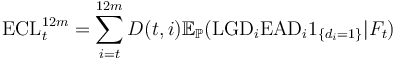# 12-month Expected Credit Losses

## Definition

12-month Expected Credit Losses in the context of IFRS 9 denote the portion of Lifetime Expected Credit Losses that represent the expected credit losses that result from default events on a financial instrument that are possible within the 12 months after the reporting date.

## Formula

Conceptually the definition is captured in the following mathematical expression$\mbox{ECL}_t^{12m} = \sum_{i=t}^{12m} D(t,i) \mathbb{E}_{\mathbb{P}} (\mbox{LGD}_{i} \mbox{EAD}_{i} 1_{ \{ d_{i} = 1 \} } | F_t )$
• Where t is the reporting date
• i denotes possible times of default / loss (normally associated with instrument cashflows) within the 12-month period
• di is the random (unknown) event of default at time i
• LGD denotes Loss Given Default
• EAD denotes Exposure at Default
• D(t,i) denotes the discount rate at time t (based on the Effective Interest Rate, for the different cashflow maturities i
• Ft denotes the subjective but Forward-Looking Information set used formulate the estimate at time t
• P denotes the subjective assignment of default probabilities to the events di

This formula captures the essence of the definition, in practice evaluation of the 12-month portion LECL may utilize a variety of simplified / approximate forms. A common simplification is the use of the concept of 1-year Probability of Default